# 编译原理: Subset Construction 子集构造法(幂集构造)(NFA转DFA)

## 简介

1. Thompson 构造法：正则表达式 RegExp -> 不确定有限状态机 NFA
2. 子集构造法：不确定有限状态机 NFA -> 确定有限状态机 DFA
3. 最小化：最小化确定有限状态机 DFA
4. 验证：DFA 转换为的等价正则表达式并验证等价

# 正文

## 示例回顾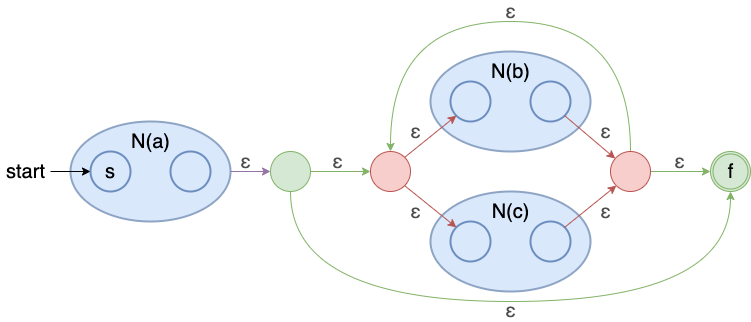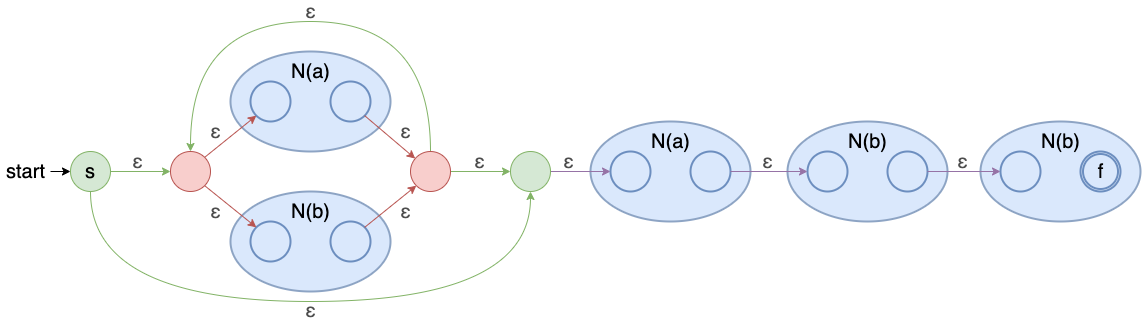## 子集构造法 Subset Construction

### 函数定义

1. ϵ − c l o s u r e ( s ) , s ∈ S N F A \epsilon-closure(s), s \in S_{NFA} 闭包
• 输入：一个状态集 s
• 输出：所有 s 中的状态可经由任意长度的 ϵ \epsilon 边能抵达的状态集合
• 补充：这边的状态指的是 NFA 中的状态
1. m o v e ( A , a ) , A ∈ S D F A , a ∈ ∑ move(A, a), A \in S_{DFA}, a \in \sum
• 输入：一个 DFA 状态(即一个 NFA 的状态的集合，后续)，一个输入字符(a)
• 输出：DFA 状态中每个 NFA 状态透过 a a 边能抵达的所有 NFA 状态的集合

### 算法过程伪代码

Subset-Construction(NFA)
let Dtran be a table
DFA_States = {ε-closure(NFA.s0)}  # DFA 状态集合的初始状态为 NFA 初始状态的闭包，并且未标记
while (exist T in DFA_States not marked) { # 存在未标记的 DFA 状态
mark T  # 标记 T，表示查过 T 状态的所有后续状态了
for (a in Σ) {
Tc = ε-closure(move(T, a)) # 找到所有输入字符对应的下一个状态
if (Tc not in DFA_States) { # 将状态加入到 DFA_States
push Tc in DFA_States & unmarked Tc
}
Dtran[T, a] = Tc
}
}
return Dtran


### 构造 Dtran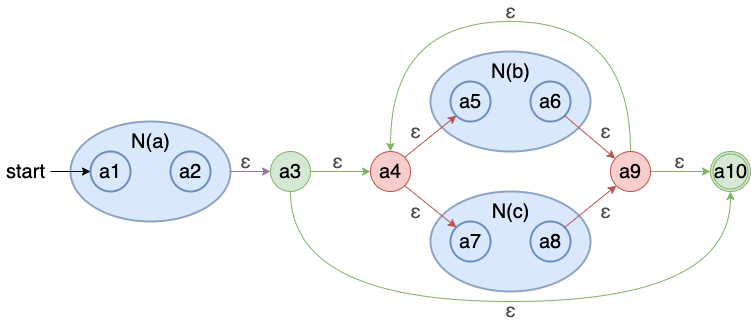1. 首先我们先求出起始 DFA 状态设为 A A
A = ε-closure({a1}) = {a1}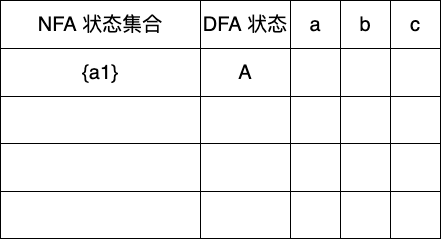1. 第二步找到未标记状态 A A ，可输入字符为 a a
Dtran[A,a] = ε-closure(move(A,a))
= ε-closure({a2})
= {a2,a3,a4,a5,a7,a10}


D t r a n [ A , a ] Dtran[A,a] 表示的集合不在 Dtran 状态表里，则记为新状态 B B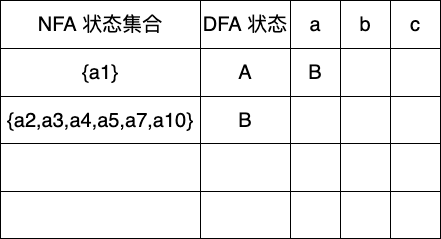1. 下一个未标记状态为 B B ，可输入字符有 b , c b, c
Dtran[B,b] = ε-closure(move(B,b))
= ε-closure({a6})
= {a4,a5,a6,a7,a9,a10}
= C

Dtran[B,c] = ε-closure(move(B,c))
= ε-closure({a8})
= {a4,a5,a7,a8,a9,a10}
= D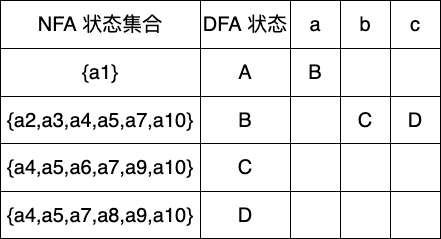1. 下一个状态 C C ，可输入字符有 b , c b, c
Dtran[C,b] = ε-closure(move(C,b))
= ε-closure({a6})
= {a4,a5,a6,a7,a9,a10}
= C

Dtran[C,c] = ε-closure(move(C,c))
= ε-closure({a8})
= {a4,a5,a7,a8,a9,a10}
= D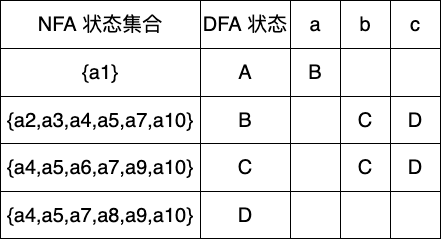1. 下一个状态 D D ，可输入字符有 b , c b, c
Dtran[D,b] = ε-closure(move(D,b))
= ε-closure({a6})
= {a4,a5,a6,a7,a9,a10}
= C

Dtran[D,c] = ε-closure(move(D,c))
= ε-closure({a8})
= {a4,a5,a7,a8,a9,a10}
= D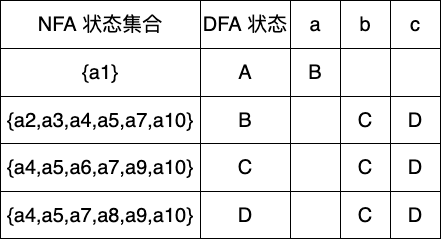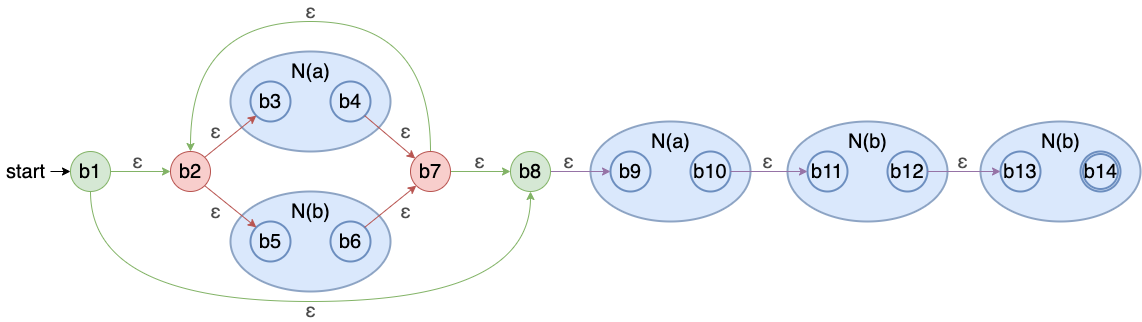1. 起始状态 A A
A = ε-closure({b1}) = {b1,b2,b3,b5,b8,b9}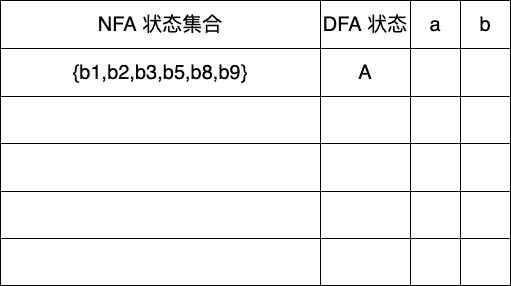1. 状态 A A ，输入 a , b a, b
Dtran[A,a] = ε-closure(move(A,a))
= ε-closure({b4,b10})
= {b2,b3,b4,b5,b7,b8,b9,b10,b11}
= 新状态 B

Dtran[A,b] = ε-closure(move(A,b))
= ε-closure({b6})
= {b2,b3,b5,b6,b7,b8,b9}
= 新状态 C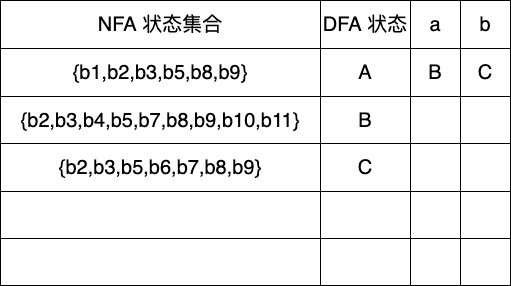1. 状态 B B ，输入 a , b a, b
Dtran[B,a] = ε-closure(move(B,a))
= ε-closure({b4,b10})
= {b2,b3,b4,b5,b7,b8,b9,b10,b11}
= B

Dtran[B,b] = ε-closure(move(B,b))
= ε-closure({b6,b12})
= {b2,b3,b5,b6,b7,b8,b9,b12,b13}
= 新状态 D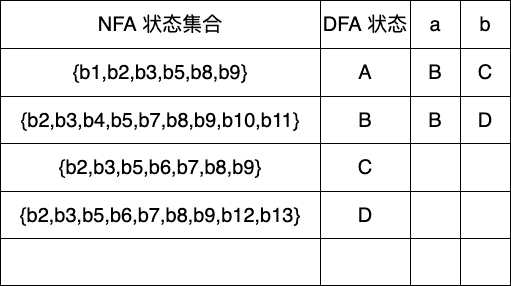1. 状态 C C ，输入 a , b a, b
Dtran[C,a] = ε-closure(move(C,a))
= ε-closure({b4,b10})
= {b2,b3,b4,b5,b7,b8,b9,b10,b11}
= B

Dtran[C,b] = ε-closure(move(C,b))
= ε-closure({b6})
= {b2,b3,b5,b6,b7,b8,b9}
= C1. 状态 D D ，输入 a , b a, b
Dtran[D,a] = ε-closure(move(D,a))
= ε-closure({b4,b10})
= {b2,b3,b4,b5,b7,b8,b9,b10,b11}
= B

Dtran[D,b] = ε-closure(move(D,b))
= ε-closure({b6,b14})
= {b2,b3,b5,b6,b7,b8,b9,b14}
= 新状态 E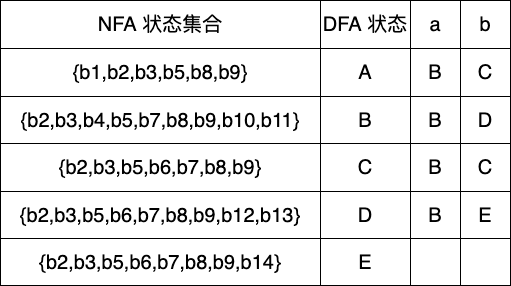1. 状态 E E ，输入 a , b a, b
Dtran[E,a] = ε-closure(move(E,a))
= ε-closure({b4,b10})
= {b2,b3,b4,b5,b7,b8,b9,b10,b11}
= B

Dtran[E,b] = ε-closure(move(E,b))
= ε-closure({b6})
= {b2,b3,b5,b6,b7,b8,b9}
= C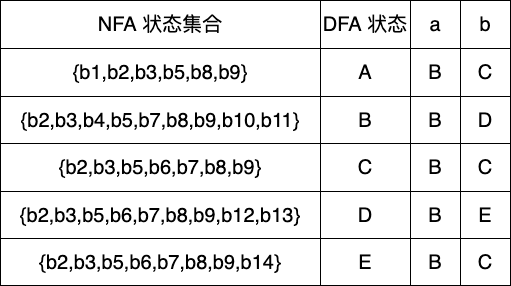### 绘制 DFA

• Dtran 表• DFA 图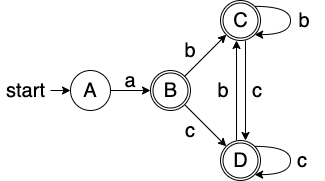• Dtran 表• DFA 图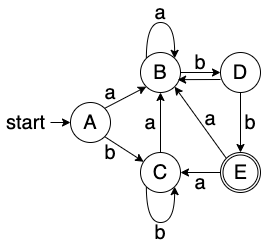# 结语

03-291436
08-09272302-081万+
03-223053
05-28
12-241万+
03-15829
11-09527
10-084343
04-041134
12-195861
01-29
03-25
10-27